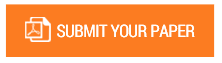Comparative Analysis of a Controller for a Magnetic Ball Levitation System

Authors : GETAHUN NIGATU AMERGA; Muluneh Hailu

Volume/Issue : Volume 6 - 2021, Issue 8 - August

Scribd : https://bit.ly/39WAi9X

Magnetic levitation system is a system that can work on the principle of magnetic attraction and repulsion to levitate an object. It presents a mathematical modelling using a Taylor series approximation method. However, the magnetic ball levitation system is mostly a non-linear and open loop unstable. So, it made the controller strategy more difficult. This thesis work introduces the control techniques of a magnetic ball levitation system depends on linear feedback which is a PID controller. Then the PID controller is more improved by an Adaptive PID with MRAC controller and was obtained a good performance characteristic. Increasing the performance of an Adaptive PID with MRAC Controller was possible with Genetic Algorithms optimization techniques by minimizing the objective function and the error. Lastly, for position control and stabilization of the magnetic ball levitation system an optimal LQR was developed and the weighting matrix Q and R based on the analytical approach was chosen as and are positive semidefinite and positive definite matrix respectively. So, the performance of an Adaptive PID with MRAC controller was more improved by a LQR controller; the performance (i.e., overshoot, Peak amplitude and settling time) obtained by a LQR controller was better when compared to a PID, an Adaptive PID with MRAC Controller and an Adaptive PID controller with GA Optimization techniques.

Keywords : Magnetic Levitation System, ProportionalIntegral-Differential Controller, Genetic Algorithms, Adaptive with MRAC Controller and Linear Quadratic Regulator Controller.

#### CALL FOR PAPERS

Paper Submission Last Date
31 - May - 2022